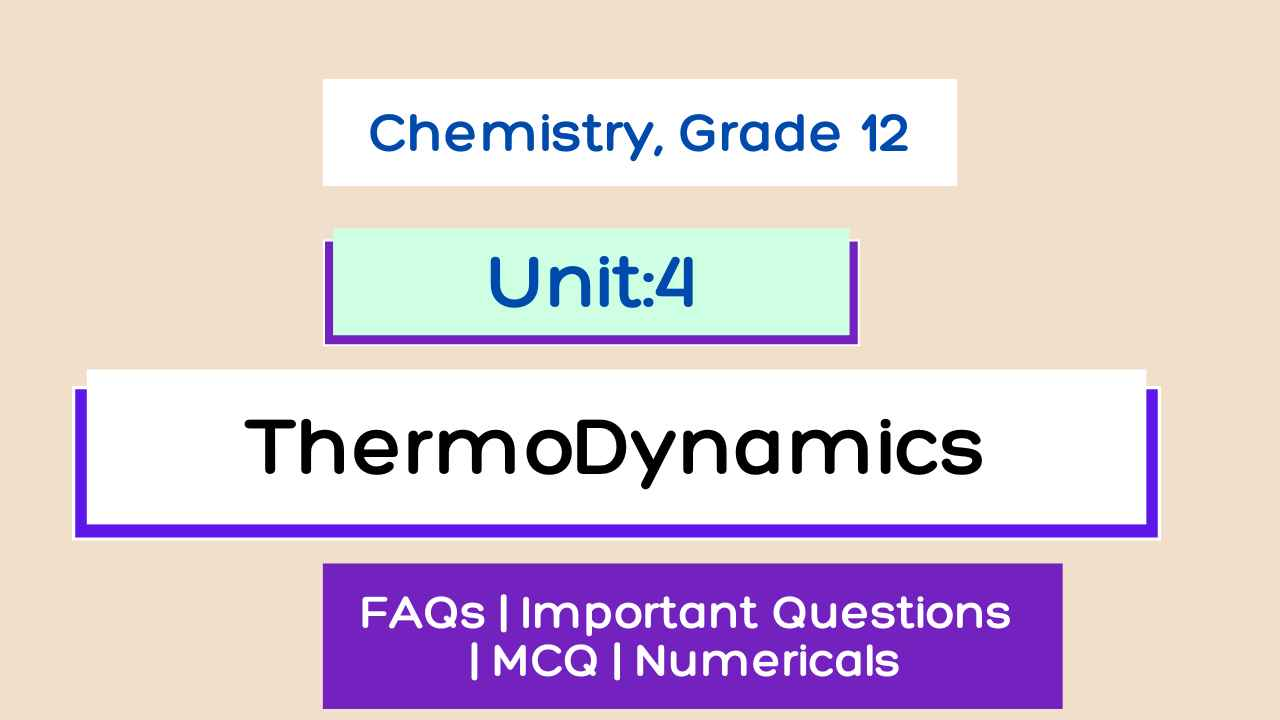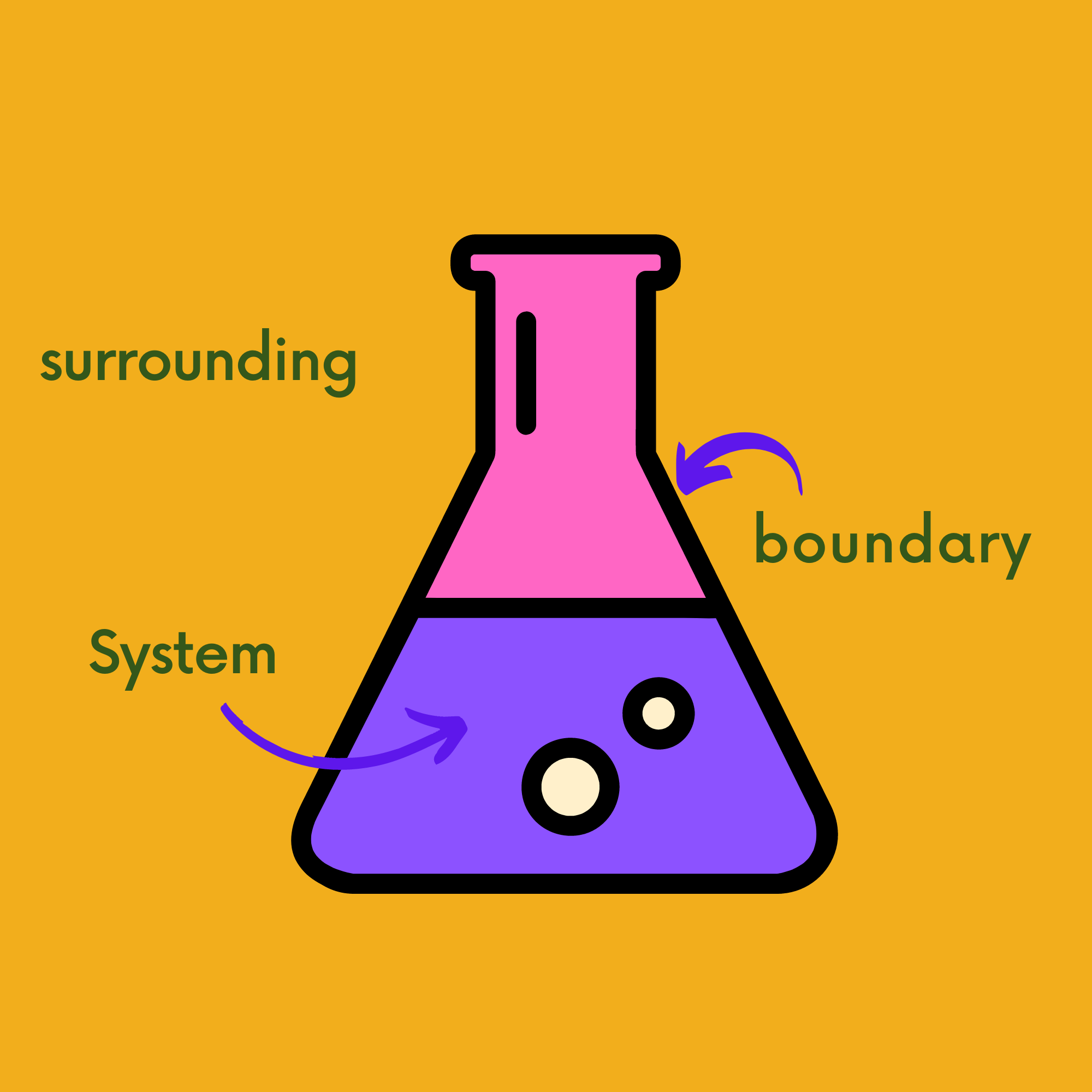# Thermodynamics Class 12 Physical Chemistry Notes

Thermodynamics Class 12 Chemistry notes With formulas, important questions, MCQs, solved numerical problems, and a PDF for NEB Chemistry exams.
Thermodynamics Course content
4.1 Define thermodynamics.
4.2 Explain the energy change in chemical reactions.
4.3 Define the terms internal energy and state function.
4.4 State and explain the first law of thermodynamics.
4.5 State and explain enthalpy and enthalpy changes in various processes (enthalpy of solution, enthalpy of formation enthalpy of combustion, and enthalpy of reaction).
4.6 Explain endothermic and exothermic processes with the help of an energy profile diagram.
4.7 State laws of thermo-chemistry and solve numerical problems related to Hess law.
4.8 Define the term entropy and spontaneity.
4.9 State and explain the second law of thermodynamics.
4.10 Define standard Gibbs free energy change of reaction by means of the equation ΔG=ΔH-TΔS.
4.11 Calculate ΔG for a reaction using the equation ΔG=ΔH-TΔS.
4.12 State whether a reaction of a process will be spontaneous by using the sign of ΔG.
4.13 Explain the relationship between ΔG and equilibrium constant.Unit 4: Thermodynamics class 12 chemistry notes Nepal

## Unit 4: Thermodynamics

Thermodynamics Class 12 Notes is given below:

Introduction of Thermodynamics

It is the branch of Science that deals with the study of the flow of heat or any form of Energy into or out of the system when it undergoes any physical or chemical transformation.

Unit 1: Volumertri Analysis Class 12 Chemistry Complete Notes

Unit 3: Chemical Kinerics  Class 12 Chemistry Complete Notes

### Some Important thermodynamics terms

#### System

A System is defined as that part of the universe that is under thermodynamics study.

#### Surroundings

The rest of the universe ( Except the system ) is known as its surroundings.

#### Boundary

A  real or imaginary line separating the surroundings and the system is called a boundary.

### Types of Thermodynamics system

1. Open System
2. Closed System
3. Isolated System

#### 1. Open system

An Open system is one that can transfer both Energy and matter to and from the surrounding.

Example: Hot water in a beaker.

The boundary of the open system isn't sealed.

#### 2. Closed system

A Closed system is one that can transfer only energy not matter to and from the surrounding.

Example: Hot water in a Hot water bag.

The boundary of the closed system is sealed.

#### 3. Isolated system

An Isolated system is one that cannot transfer both Energy and matter to and from the surrounding.

The boundary of the Isolated system is closed and insulated.Pic: System, surrounding and boundary in thermodynamics system

### Phase In Thermodynamics

1. Homogeneous System
2. Heterogeneous System

#### 1. Homogeneous System

A Homogeneous System is one that is uniform throughout and it contains only one phase.

Examples: A pure solid or liquid or gas, a mixture of gases, etc.

#### 2. Heterogeneous System

A Heterogeneous System is one that is not uniform throughout and it contains more than one phase.

Examples: Ice Water, CH3Cl Water, etc.

### Intensive and Extensive Properties

#### Intensive properties

Those Properties that don't depend upon the quantity of matter are called Intensive properties.

Example: Boiling point of water, Density of water or liquid, etc.

#### Extensive properties

Those properties which depend upon the quantity of matter are called Extensive properties.

Example: Volume, Weight, etc.

### State Function or State Variables

A thermodynamics system is said to be in a state where all of its Fundamental properties are fixed.

The Fundamental properties which defined a state of a system are pressure, temperature, number of moles ( its composition ), and Volume.

The change in the value of these properties alters the state of a system.

These Properties are called State functions or State Variables.

For a pure substance, its composition is fixed as it is 100%.

If a mole of pure gas is taken the remaining state variable are related to one another by an algebraic equation called Equation of State.

PV = RT
where R is constant( Universal Gas Constant )

If P and T are Specified V is automatically fixed.

so, Pressure(P) and Time(T) are Called Independent State Variables as their Volume is Depend upon Pressure and Temperature.

### Process

When a system undergoes from one state( initial ) to another state ( final ) then the Operation is called Process.

There are different thermodynamic processes given:
1. Isothermal Process
3. Isobaric Process
4. IsoChoric Process
5. Cyclic process

Getting Info...